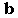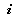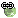Modern Maths

Equation 1
Human = eat + sleep + work + enjoy

Donkey = eat + sleep

Therefore,

Human = Donkey + work + enjoy

if, Human - enjoy = Donkey + work

In other words,

Human that don't know enjoy = Donkey that work
(Equation 1)

Equation 2

Men = eat + sleep + earn money

Donkeys = eat + sleep

Therefore,
Men = Donkeys + earn money
(Equation 2)

If Men - earn money = Donkeys

In other words,

Men that don't earn money = Donkeys
(Equation 3)

Equation 3

Women = eat + sleep + spend

Donkeys = eat + sleep

Therefore,
Women = Donkeys + spend
(Equation 4)

If, Women - spend = Donkeys

In other words,

Women that don't spend = Donkeys
(Equation 5)

To Conclude:

From Equation 3 and Equation 5
Men that don't earn money = Women that don't spend
So, Men earn money not to let women become Donkeys!
(Postulate 1)

Women spend not to let men become Donkeys!
(Postulate 2)

From Equation 2 + Equation 4, we have Men + Women = Donkeys + earn money + Donkeys + spend
From Postulates 1 and
2, we can conclude

Man + Woman = 2 Donkeys

And the Donkeys live happily ever after!

Labels:

var a = 0; if(a == 0) {document.write('No comments yet.');} else if(a == 1){document.write('1 comment so far');} else {document.write(a+' comments');}Convert to boldConvert to italicConvert to link